## Functions Exercise 1b Solutions

Functions Exercise 1b

The famous mathematician ” Lejeune Dirichlet”  defined a function.

Function: A variable is a symbol which represents any one of a set of numbers, if two variables x and y so related that whenever a value is assigned to x there is autometically assigned by some rule or correspondence a value to y, then we say y is a function of x.

Chapter 1 Functions Exercise 1b Solutions for inter first year  students, prepared by Mathematics expert of www.basicsinmaths.com

## Functions Exercise 1b

Exercise 1(b) Solutions

### I.

##### 1.If f (x) = ex and g(x) =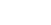, then show that f og = gof and f-1 = g-1

Sol:

Given     f (x) = ex and g(x) =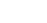(fog) (x) = f (g (x))

= f ()

=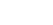= x ————- (1)

(gof) (x) = g (f (x))

= g (ex)

=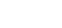= x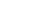= x ————- (2)

From (1) and (2)  f og = gof

let y = f(x)

x = f-1(y)

y = ex

x =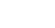f-1 (x) =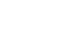let g(x) = z

x = g-1(z)

z =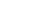x = ez

g-1 (x)  = ex

##### 2. If f (y) =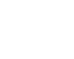,  g (y) =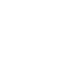then show that fog(y) = y

Sol:

Given f (y) =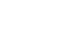,  g (y) =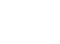Now

fog(y) = f(g(y))∴ fog(y) = y

##### (i)  (fog) (x)    (ii) (gof) (x)       (iii) (fof) (0)      (iv) go(fof)(3)

Sol:

Given f: R ⟶ R is, g: R ⟶ R is defined by f(x) = 2x2 + 3 ang g (x) = 3x – 2

(i)  (fog) (x) = f(g(x))

= f(3x – 2)

= 2 (3x – 2)2 + 3

= 2 (9x2 – 12x + 4) + 3

= 18 x2 – 24x + 8 + 3

= 18 x2 – 24x + 11

∴ (fog) (x) = 18 x2 – 24x + 11

(ii)  (gof) (x) = g (f (x))

= g (2x2 + 3)

= 3(2x2 + 3) – 2

= 6x2 + 9 – 2

= 6 x2 + 7

∴ (gof) (x) = 6 x2 + 7

 PDF Files || Inter Maths 1A &1B || (New)6th maths notes|| TS 6 th class Maths ConceptTS 10th class maths concept (E/M)

(iii)  (fof) (0) = f (f (0))

= f (2(0)2 + 3)

= f (2(0) + 3)

= f (3)

= 2 (3)2 + 3

= 2 (9) + 3

= 18 + 3 = 21

(iv)  go(fof) (3) = go (f (f (3)))

= go (f (2 (3)2 + 3))

= go (f (21))

= g (f (21))

= g (2 (21)2 + 3))

= g (2 (441) + 3))

= g (882 + 3)

= g (885)

= 3 (885) – 2

= 2655 – 2

= 2653

∴ go(fof) (3) = 2653

Functions Exercise 1b

Ts Inter Maths IA Concept

##### (i) (fof) (x2 + 1)    (ii) (fog) (2)        (iii) (gof) (2a – 3)

Sol:

Given f: R ⟶ R; g: R ⟶ R is defined by f(x) = 3x – 1, g (x) = x2 + 1

(i) (fof) (x2 + 1) = f (f (x2 + 1))

= f (3 (x2 + 1)– 1)

= f (3x2 + 3– 1)

= f (3x2 + 2)

= 3(3x2 + 2) – 1

= 9x2 + 6 – 1

= 9x2 + 5

(ii) (fog) (2) = f (g (2))

= f (22 + 1)

=f (4 + 1)

= f (5)

= 3(5) – 1

= 15 – 1

= 14

(iii) (gof) (2a – 3) = g (f (2a – 3))

= g (3(2a – 3) – 1)

= g (6a – 9 – 1)

= g (6a – 10)

= (6a – 10)2 + 1

= 362 – 120a + 100 + 1

= 362 – 120a + 101

##### 5. If f(x) =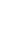and g(x) =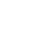for all x ∈ (0, ∞) then find (gof) (x)

Sol:

Given f(x) =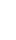and g(x) =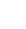for all x ∈ (0, ∞)

(gof) (x) = g (f (x))

= g (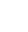)

=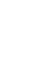∴ (gof) (x) =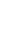##### 6. If f(x) = 2x – 1 and g(x) =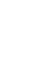for all x ∈ R then find (gof) (x)

Sol:

Given f(x) = 2x – 1 and g(x) =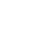for all x ∈ R∴ gof(x) = x

##### 7. If f (x) = 2, g (x) = x2 and h(x) = 2x ∀ x ∈ R, then find (fo(goh)) (x)

Sol:

Given f (x) = 2, g (x) = x2 and h(x) = 2x ∀ x ∈ R

(fo(goh)) (x) = fo (g (h (x))

= fo g(2x)

= f (g(2x))

= f((2x)2)

= f(4x2)

= 2

∴ (fo(goh)) (x) = 2

Functions Exercise 1b Solutions

#### 8. Find the inverse of the following functions

(i) a, b ∈ R, f: R ⟶ R defined by f(x) = ax + b, (a ≠ 0)

Given a, b ∈ R, f: R ⟶ R defined by f(x) = ax + b

Let y = f(x)

x = f-1(y)

now y = ax + b

ax = y – b

x =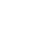f-1(y) =∴   f-1(x) =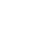(ii) f: R ⟶ (0, ∞) defined by f(x) = 5x

Let y = f(x)

x = f-1(y)

now y = 5x

x =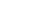f-1(y) =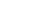∴   f-1(x) =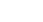(iii) f: (0, ∞) ⟶ R defined by f(x) =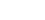Let y = f(x)

x = f-1(y)

now y =x = 2y

f-1(y) = 2y

∴   f-1(x) = 2x

##### 9. If f(x) = 1 + x + x2 + … for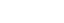, then show that f-1 (x) =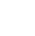Sol:

Given, f(x) = 1 + x + x2 + … for

1 + x + x2 + … is an infinite G.P

a = 1, r = x

S =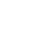=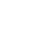Now

f (x) =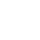Let y = f(x)

x = f-1(y)

now y =1 – x =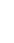x = 1 –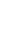=f-1(y) =∴   f-1(x) =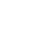##### 10 . If f: [1, ∞) ⟶ [1, ∞) defined by f (x) = 2x (x – 1) then find f-1 (x)

Sol:

Given f: [1, ∞) ⟶ [1, ∞) defined by f (x) = 2x (x – 1)

Let y = f(x)

x = f-1(y)

now y = 2x (x – 1)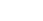= x (x – 1)

x2 – x =x2 – x –= 0Functions Exercise 1b Solutions

### II.

#### 1. If f (x) =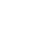, x ≠ ± 1, then verify (fof-1) (x) = x

Sol:

Given f (x) =, x ≠ ± 1

Let y = f(x)

x = f-1(y)

now y =y (x + 1) = x – 1

xy + y = x – 1

1 + y = x – xy

1 + y = x (1 – y)

x =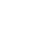f-1(y) =∴   f-1(x) =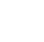Now##### 2.  If A = {1, 2, 3}, B = {α, β, γ}, C = {p, q, r} and f: A ⟶ B; g: B ⟶ C is defined by f = {(1, α), (2, γ), (3, β)}, g = {(α, q), (β, r), (γ, p)}, then show that f and g are bijective functions and (gof)-1 = f-1og-1

Sol:

Given A = {1, 2, 3}, B = {α, β, γ}, C = {p, q, r} and f: A ⟶ B; g: B ⟶ C is defined by f = {(1, α), (2, γ), (3, β)}, g = {(α, p), (β, r), (γ, p)}

A = {1, 2, 3}, B = {α, β, γ}

f: A ⟶ B; and f = {(1, α), (2, γ), (3, β)}

⟹ f (1) = α; f (2) = γ; f (3) = β

⟹    Every element of set A has a unique image in set B

f is injection (one – one)

range of f = codomain of f

⟹ f is surjection (on to)

∴ f is bijective

B = {α, β, γ}, C = {p, q, r}

g: B ⟶ C is defined by g = {(α, q), (β, r), (γ, p)}

⟹ g (α) =q; g (β) = r; g (γ) = p

⟹    Every element of set B has a unique image in set C

g is injection (one – one)

range of g = codomain of g

⟹ g is surjection (on to)

∴ g is bijective

Now

f = {(1, α), (2, γ), (3, β)}, g = {(α, q), (β, r), (γ, p)}

gof = {(1, q), (2, p), (3, r)

(gof)-1 = {(q, 1), (p, 2), (r, 3)} ———– (1)

f-1 = {(α, 1), (γ, 2), (β, 3)

g-1 = {(q, α), (r, β), (p, γ)}

f-1og-1 = {(q, 1), (p, 2), (r, 3)} ———– (2)

From (1) and (2)

(gof)-1 = f-1og-1

##### (i) (gof-1) (2)           (ii) (gof) (x – 1)

Sol:

Given f: R ⟶ R; g: R ⟶ R is defined by f (x) = 3x – 2 and g(x) = x2 + 1

Let y = f(x)

x = f-1(y)

now y = 3x – 2

3x = y + 2

x =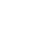f-1(y) =f-1(x) =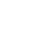∴ (gof-1) (2)   =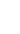(ii)  (gof) (x – 1) = g (f (x – 1))

= g (3(x – 1) – 2)

= g (3x – 3 – 2)

= g (3x – 5)

= (3x – 5)2 + 1

= 9x2 – 30x + 25 + 1

= 9x2 – 30x + 26

∴(gof) (x – 1) = 9x2 – 30x + 26

##### 4. Let f = {(1, a), (2, c), (4, d), (3, b)} and g-1 = {(2, a), (4, b), (1, c), (3, d)}, then show that (gof)-1 = f-1og-1

Sol:

Given f = {(1, a), (2, c), (4, d), (3, b)} and g-1 = {(2, a), (4, b), (1, c), (3, d)}

⟹ f -1 = {(a, 1), (c, 2), (d, 4), (b, 3)} and g = {(a, 2), (b, 4), (c, 1), (d, 3)}

Now gof = {(1, 2), (2, 1), (4, 3), (3, 4)}

(gof)-1 = {(2, 1), (1, 2), (3, 4), (4, 3)} ———(1)

g-1 = {(2, a), (4, b), (1, c), (3, d)} ; f -1 = {(a, 1), (c, 2), (d, 4), (b, 3)}

f-1og-1 = {(2, 1), (1, 2), (3, 4), (4, 3)} ———(2)

from (1) and (2)

(gof)-1 = f-1og-1

##### 5. If f: R ⟶ R; g: R ⟶ R is defined by f (x) = 2x – 3 and g(x) = x3 + 5 then find (fog)-1 (x)

Sol:

Given R ⟶ R; g: R ⟶ R is defined by f (x) = 2x – 3 and g(x) = x3 + 5

(fog) (x) = f (g(x))

= f (x3 + 5)

= 2 (x3 + 5) – 3

= 2 x3 + 10 – 3 = 2x3 + 7

Let y = (fog) (x)

⟹ x = (fog)-1(y)

y = 2x3 + 7

2x3 = y – 7

x3 =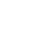x =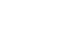(fog)-1(y)=(fog)-1(x)=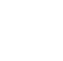##### 6. Let f(x) = x2, g(x) = 2x then solve the equation (fog) (x) = (gof) (x)

Sol:

Given f(x) = x2, g(x) = 2x

(fog) (x) = f(g(x))

= f (2x)

= (2x)2

= 22x

(gof) (x) = g(f(x))

= g (x2)

=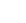Now

(fog) (x) = (gof) (x)

⟹ 22x =2x = x2   [∵ if am = an , then m = n]

x2 – 2x = 0

⟹ x (x – 2) = 0

⟹x = 0 or x – 2 = 0

∴ x = 0 or x = 2

##### 7.  If f (x) = , x ≠ ±1 then find (fofof) (x) and (fofofof) (x)

Sol:

Given f (x) =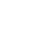, x ≠ ±1PDF Files || Inter Maths 1A &1B || (New)6th maths notes|| TS 6 th class Maths ConceptTS 10th class maths concept (E/M)Ts Inter Maths IA ConceptTS 10th Class Maths Concept (T/M)

##### Visit my Youtube Channel: Click on Below Logo